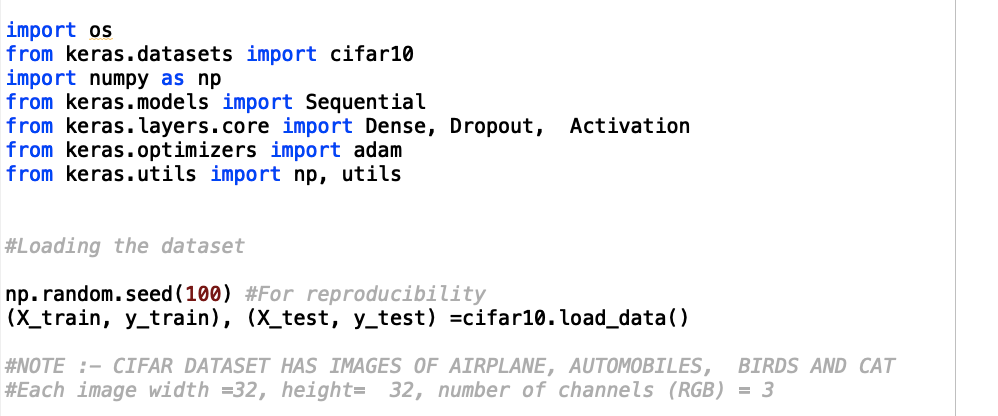# Major Steps used in Deep Learning Model

Original article can be found here (source): Deep Learning on Medium# Major Steps used in Deep Learning Model

What is the Sequential model and Functional API in Keras? What is the loss function, types of it? what are the optimizer and its type?

If you’ve not gone through, Understand the concepts of Deep learning before this.

1. Define the model. Sequential model or functional model –
2. Compile the model and here you add loss function and optimizer before calling compile() function on the model
3. Fit the model with the training dataset. And Test the model on test data by calling fit() function model
4. Make a prediction — here you make a prediction by calling evaluate or predict() function

There are 8 steps in Deep Learning process:-